Subject:
Geometry
Material Type:
Lesson Plan
Level:
Middle School
6
Provider:
Pearson
Tags:
• Measurements and Data
• Parallelograms
• Triangles
Language:
English
Media Formats:
Interactive, Text/HTML

# Analyzing The Formula of A Triangle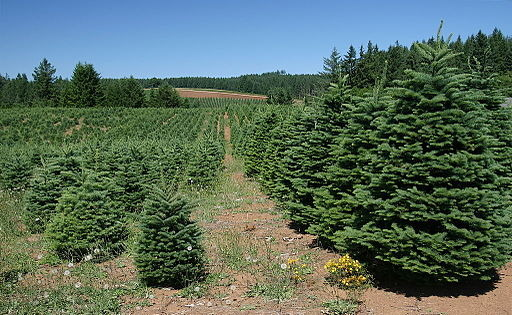# Lesson Overview

Students find the area of a triangle by putting together a triangle and a copy of the triangle to form a parallelogram with the same base and height as the triangle. Students also create several examples of triangles and look for relationships among the base, height, and area measures. These activities lead students to develop and understand a formula for the area of a triangle.

# Key Concepts

To find the area of a triangle, you must know the length of a base and the corresponding height. The base of a triangle can be any of the three sides. The height is the perpendicular distance from the vertex opposite the base to the line containing the base. The height can be found inside or outside the triangle, or it can be the length of one of the sides.

You can put together a triangle and a copy of the triangle to form a parallelogram with the same base and height as the triangle. The area of the original triangle is half of the area of the parallelogram. Because the area formula for a parallelogram is A = bh, the area formula for a triangle is A = $\frac{1}{2}$bh.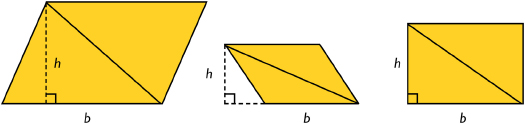# Goals and Learning Objectives

• Develop and explore the formula for the area of a triangle.

# Lesson Guide

Have students work in pairs to discuss the statements.

ELL: Students may have difficulty determining the perpendicular height of a triangle, especially when the height needs to be sketched outside of the triangle. Use the edges of an index card to help students get a better idea of how to determine the perpendicular height. Model this activity for students. The index card is aligned with the base and then shifted to the left (or right) until the vertex opposite the base touches the index card. This clearly shows that the height is perpendicular to the base.

# Mathematics

Have students look at the triangles. Make sure they understand what the base and height of a triangle are. Point out that the height can be inside the triangle, outside the triangle, or one of the sides of the triangle.

## Opening

Discuss the following statements.

• The base of a triangle can be any of the three sides.
• The height of a triangle is the perpendicular distance from the base to the vertex opposite the base.
• As shown in the diagram, the height can be inside or outside the triangle, or it can be one of the sides.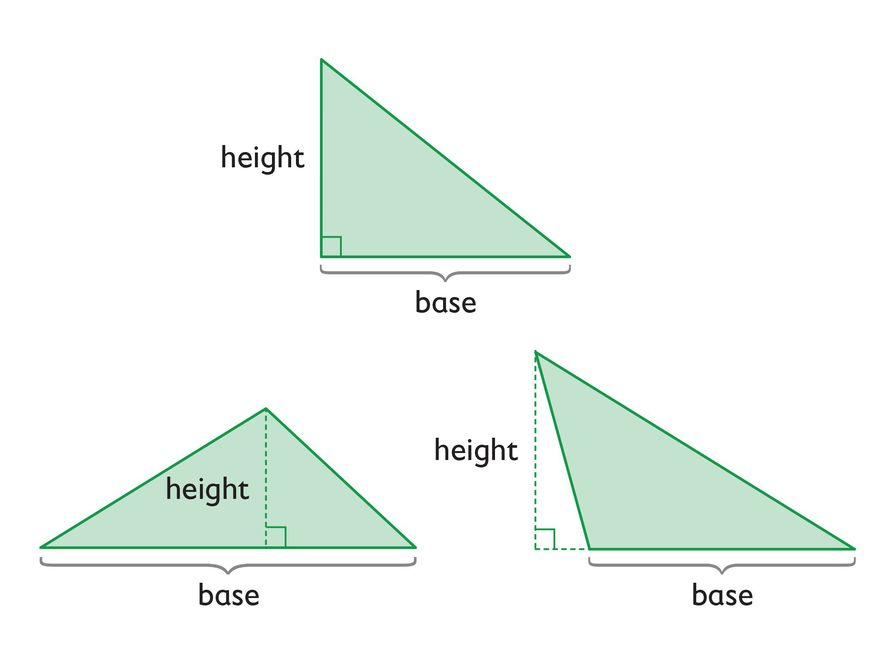# Lesson Guide

Partners should discuss how they can arrange a triangle and its copy to form a parallelogram. Students should recognize that the area of each triangle is half the area of the parallelogram made from two of the same-size triangles. Since the area of the parallelogram is A = bh, the area of the triangle is A = $\frac{1}{2}$bh.

SWD: Some students may not immediately see how to arrange the triangles into a parallelogram. If students are struggling to the point of frustration, model how to use two triangles to create a small parallelogram. Allow students to use paper cutouts if needed.

# Introduction to Triangles

Can you take any triangle, copy it, and then combine the two triangles so that they form a parallelogram?

Try it with triangles like the ones in the diagram.

• What do your results tell you about the area of a triangle?
• Write a formula for the area of a triangle.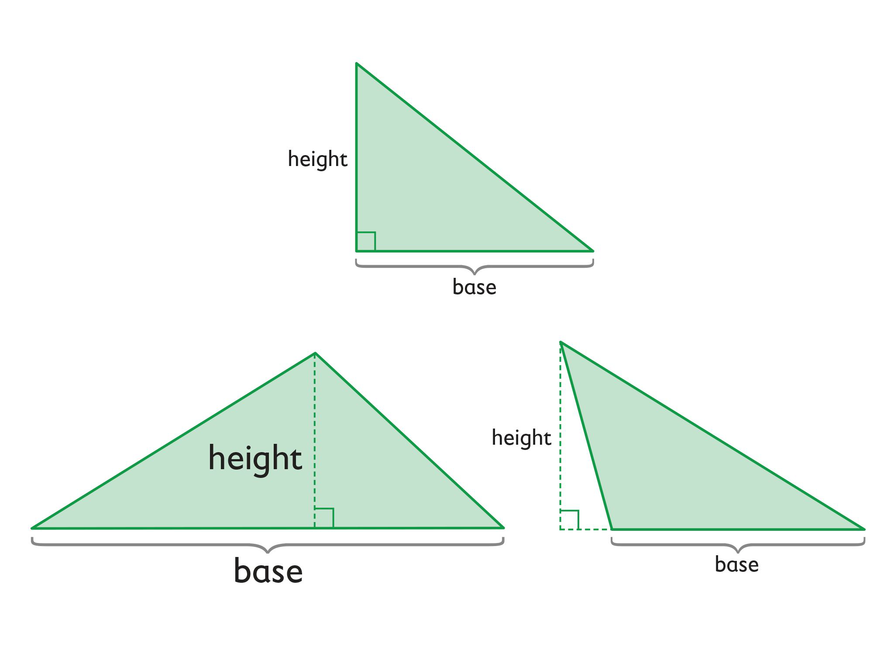# Lesson Guide

Discuss the Math Mission. Students will explore the formula for the area of a triangle.

SWD: It may be challenging for some students to remember which formulas correspond for each shape. Have students create resources for themselves to refer to throughout the unit (e.g., note cards, digital sticky notes, anchor charts, their notebook) that include the shape's name, the formula for area, and an image that represents the shape.

## Opening

Explore the formula for the area of a triangle.

# Lesson Guide

Have partners answer the questions and work on the presentation together.

SWD: Assign students concrete lengths for base and heights. If students are using cutouts in addition to the interactive, have them label the cutouts with the resulting areas. This will help them to understand the relationship between the areas of the triangles and the combined total of the areas as the area of the original polygon.

# Mathematical Practices

Mathematical Practice 7: Look for and make use of structure.

Identify students who understand how the operations in the formula impact the answer (i.e., increasing a factor increases the product; decreasing a factor decreases the product; or keeping the factors the same does not change the product).

Mathematical Practice 8: Look for and express regularity in repeated reasoning.

Watch for students who, through repeated trials, reason that if one variable is constant and the other variable increases (or decreases), then the area increases (or decreases) as well.

Look for students who, through repeated trials, reason that if both variables remain constant, the area remains constant as well.

# Interventions

Student has difficulty getting started.

• What does it mean to keep the height or base constant?
• Remember that if the vertex moves parallel to the base, then it remains the same distance from the base.
• How can you move the vertex parallel to the base?

Student changes the variable only one way, just increasing (or decreasing) its value.

• Can you decrease (or increase) the height? What happens?

Student works unsystematically.

• Can you organize the information for the base, height, and area in a table?
• Look at the values in the rows of your table. How are they related?

Student has a correct solution.

• How did you reach your conclusion?
• Explain how the Triangle interactive helped you reach a conclusion.

• If you keep the height and base constant and move the vertex parallel to the base, the area remains constant.
• If you keep the base constant and increase (or decrease) the height, then the area increases (or decreases) as well.
• Facts will vary.

# Explore the Area of Triangles

The formula for the area of a triangle is
area = $\frac{1}{2}$ •  base • height, or A = $\frac{1}{2}$bh

Use the Triangle interactive to explore the area of a triangle. Move the vertices of the triangle and explore what happens to the area.

• What happens if you keep the height and base constant and move the vertex parallel to the base?
• What happens if you keep the base constant and change the height?
• Try to discover one more interesting fact about a triangle and its area that you can share with the class.

INTERACTIVE: Triangle

## Hint:

• How does knowing the formula for the area of a parallelogram help you understand the formula for the area of a triangle?
• There are two variables, base and height, that determine the area of a triangle. A triangle also has angle measures and side lengths for the two “non-base” sides. Try experimenting with all of these measures.

# Preparing for Ways of Thinking

As students work on the problems, look for examples to share in the Ways of Thinking discussion:

• Students who understand that if the height and base are constant and the vertex moves in a parallel line, the area remains constant
• Students who recognize that if one variable is constant, increasing (or decreasing) the other variable also increases (or decreases) the area
• Students who recognize that if the base (or height) increases (or decreases) by a factor, then the area increases (or decreases) by the same factor
• Students who do not see a relationship between height, base, and area
• Students who recognize the relationship between the formula for the area of a parallelogram and the area of a triangle

# Challenge Problem

• As the vertex of the triangle slides along the line, the area will stay the same.
• Possible answer: The base of the triangle is always the same. Because the vertex stays on a line that is parallel to the base, the height will always be the same too. Because the area of the triangle depends only on the base and the height, the area will not change.

# Prepare a Presentation

• Select one of your conclusions about what happens to the area of a triangle when you change one or more variables.
• Be prepared to demonstrate your conclusion using the Triangle interactive, and to support your thinking mathematically.

# Challenge Problem

Suppose the base of a triangle lies on one of two parallel lines, and the vertex opposite the base lies on the other parallel line.

• If you slide the vertex along the line, what do you think will happen to the area of the triangle? Use the Triangle interactive to test your prediction.

INTERACTIVE: Triangle

# Mathematics

Have students share their work. Be sure to show the work of students who had trouble and those who developed incorrect conclusions, as all students can benefit from the discussion. Use the Triangle interactive to test the statements that students generated to verify them.

Have students who did the Challenge Problem share their thinking. Ask class members to critique whether their reasoning makes sense.

ELL: As with other discussions, encourage ELL students to use the academic vocabulary they have learned. Introduce new vocabulary as needed. As they participate in the discussion, be sure to monitor for knowledge of the topic.

# Make Connections

• Take notes about your classmates’ conclusions concerning what happens to the area of a triangle when you change one or more variables.

## Hint:

• What surprised you in your exploration of the area of a triangle?
• How do your conclusions about the area of a triangle compare with those of other presenters?

# Lesson Guide

Have students work on this problem on their own.

# Mathematics

As you review the answers to the problem, encourage students to share their solution methods. Identify the following ways of thinking and correct any misconceptions:

• Students who correctly use the area formulas to calculate the areas
• Students who do not use the formula for the area of a trapezoid for the first figure, but instead, find the area of the triangle and the area of the rectangle and add the two areas
• [common error] Students who use a base of 5 in., instead of 15 in., when calculating the area of the trapezoid (i.e., fail to add 5 in. + 10 in. to find the length of the longer base)

• The area of the trapezoid is 100  in2.

# Area of Trapezoid

• Find the area of this trapezoid.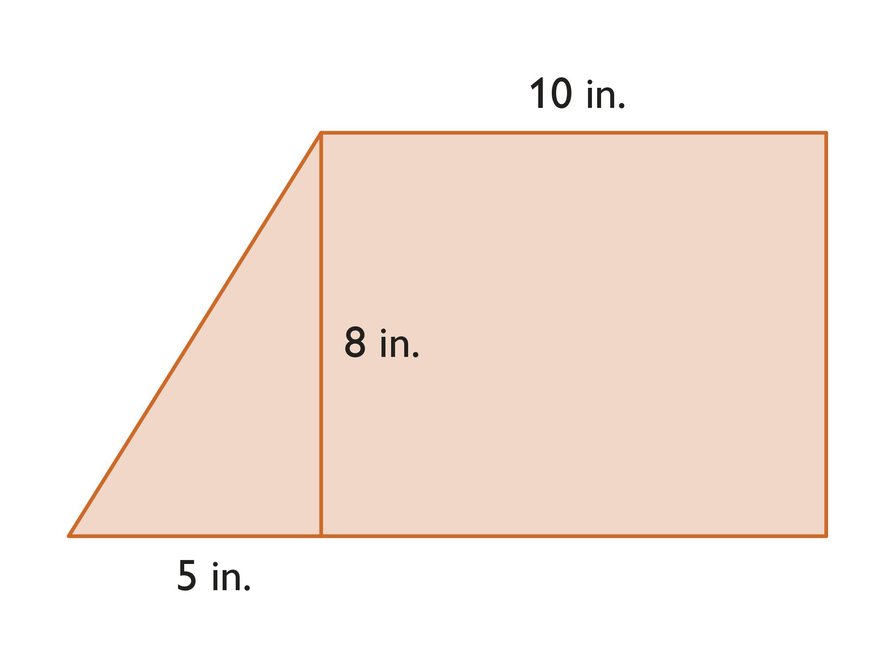# Lesson Guide

Have students work on this problem on their own.

# Mathematics

As you review the answers to these problems, encourage students to share their solution methods. Identify the following ways of thinking and correct any misconceptions:

• [common error] Students who do not use the correct base to find the area of the triangle
• Students who do not label their answers using square units

• The area of the triangle is 17.2961 cm2.

# Area of Triangle

• Find the area of this triangle.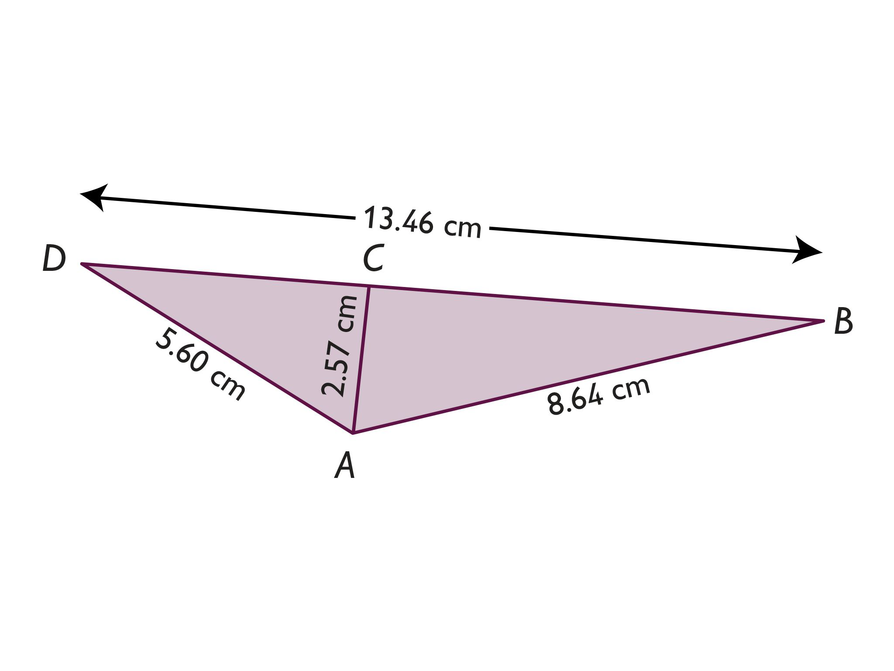# Mathematics

Have pairs quietly discuss how they can find the area of a parallelogram, a trapezoid, and a triangle if they know the formula for the area of a rectangle.

As student pairs work together, listen for students who may still have misconceptions so you can address them in the class discussion.

After a few minutes, discuss the summary as a class. Review the following points:

• You can move parts of a parallelogram around to make a rectangle. Once you have formed a rectangle, you can find its area. The formula for the area of a parallelogram is A = bh, where b is the base and h is the height.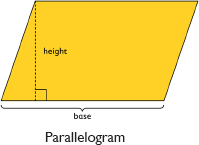• You can make a copy of a trapezoid, put the two trapezoids together to make a parallelogram, find the area of the parallelogram, and take half of that area to get the area of the original trapezoid. The formula for the area of a trapezoid is A = $\frac{1}{2}$(b1 + b2)h, where b1 is one base, b2 is the other base, and h is the height.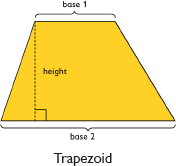• You can copy a triangle and put the triangle and its copy together to form a parallelogram. The area of the triangle is half the area of the parallelogram. The formula for the area of a triangle is A = $\frac{1}{2}$bh.ELL: Write the key points on a poster so that students can refer back to them throughout the module. When working with ELLs, provide supplementary materials, such as graphic organizers to illustrate new concepts and vocabulary necessary for mathematical learning. Have students record all information in their Notebook.

# Area Formulas

• The area of a rectangle is equal to its base times its height.
A = bh
• The area of a parallelogram is equal to its base times its height.
A = bh
• The area of a trapezoid is equal to one half times the sum of the bases times the height.
A = $\frac{1}{2}$(b1 + b2)h
• The area of a triangle is equal to one half the base times the height.
A = $\frac{1}{2}$bh

## Hint:

Can you:

• Calculate the area of a triangle, parallelogram, or trapezoid given the values of the base(s) and height?
• Calculate the height of a triangle, parallelogram, or trapezoid given the values of the base(s) and area?